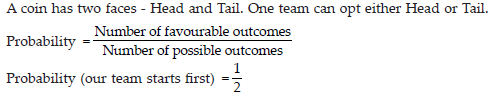Data Handling (Maths) Class 7 - NCERT Questions
Q 1.

Find the range of heights of any ten students of your class.

SOLUTION:

Let the heights (in cm) of 10 students of our class be 125, 129, 131, 132, 134, 136, 139, 142, 144, 146 Highest observation = 146,
Lowest observation = 125
Range = Highest observation – Lowest observation = (146 – 125) cm = 21 cm

Q 2.

Organise the following marks in a class assessment, in a tabular form. 4, 6, 7, 5, 3, 5, 4, 5, 2, 6, 2, 5, 1, 9, 6, 5, 8, 4, 6, 7
(i) Which number is the highest?
(ii) Which number is the lowest?
(iii) What is the range of the data?
(iv) Find the arithmetic mean.

SOLUTION: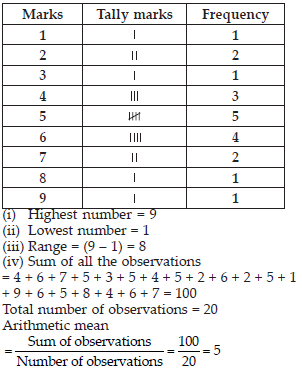Q 3.

Find the mean of the first five whole numbers.

SOLUTION: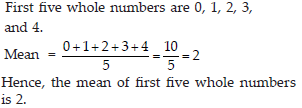Q 4.

A cricketer scores the following runs in eight innings:
58, 76, 40, 35, 46, 45, 0, 100.
Find the mean score.

SOLUTION: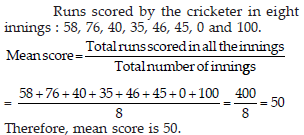Q 5.

Following table shows the points of each player scored in four games: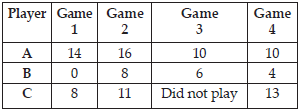Now answer the following questions: (i) Find the mean to determine A’s average number of points scored per game.
(ii) To find the mean number of points per game for C, would you divide the total points by 3 or by 4? Why?
(iii) B played in all the four games. How would you find the mean?
(iv) Who is the best performer?

SOLUTION: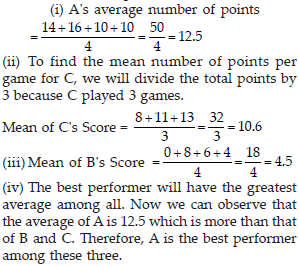Q 6.

The marks (out of 100) obtained by a group of students in a Science test are 85, 76, 90, 85, 39, 48, 56, 95, 81 and 75. Find the:
(i) Highest and the lowest marks obtained by the students.
(ii) Range of the marks obtained.
(iii) Mean marks obtained by the group.

SOLUTION: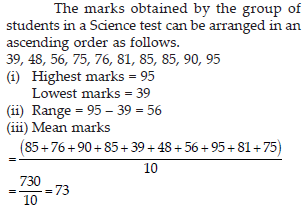Q 7.

The enrolment in a school during six consecutive years was as follows:
1555, 1670, 1750, 2013, 2540, 2820 Find the mean enrolment of the school for this period.

SOLUTION: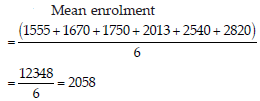Q 8.

The rainfall (in mm) in a city on 7 days of a certain week was recorded as follows: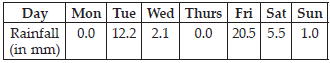(i) Find the range of the rainfall in the above data.
(ii) Find the mean rainfall for the week.
(iii) On how many days was the rainfall less than the mean rainfall?

SOLUTION: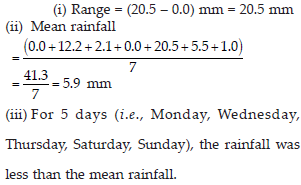Q 9.

The heights of 10 girls were measured in cm and the results are as follows:
135, 150, 139, 128, 151, 132, 146, 149, 143, 141.
(i) What is the height of the tallest girl?
(ii) What is the height of the shortest girl?
(iii) What is the range of the data?
(iv) What is the mean height of the girls?
(v) How many girls have heights more than the mean height?

SOLUTION:Q 10.

The scores in Mathematics test (out of 25) of 15 students are as follows: 19, 25, 23, 20, 9, 20, 15, 10, 5, 16, 25, 20, 24, 12, 20
Find the mode and median of this data. Are they same?

SOLUTION:

Arranging the given scores in ascending order, we have
5, 9, 10, 12, 15, 16, 19, 20, 20, 20, 20, 23, 24, 25, 25 Mode of given data is that value of observation which occurs for the most number of times i.e., 20. Median of the given data is the middle observation when the data is arranged in ascending order \ 8th term = 20
Hence, mode of data = median of data.

Q 11.

The runs scored in a cricket match by 11 players are as follows:
6, 15, 120, 50, 100, 80, 10, 15, 8, 10, 15 Find the mean, mode and median of this data. Are the three same?

SOLUTION: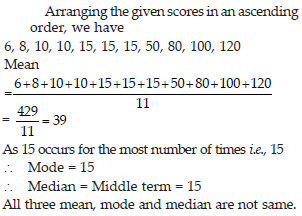Q 12.

The weights (in kg.) of 15 students of a class are:
38, 42, 35, 37, 45, 50, 32, 43, 43, 40, 36, 38, 43, 38, 47
(i) Find the mode and median of this data.
(ii) Is there more than one mode?

SOLUTION:

Arranging the given weights in ascending order, we have 32, 35, 36, 37, 38, 38, 38, 40, 42, 43, 43, 43, 45, 47, 50
(i) Mode = 38 and 43
Median = Middle term = 40
(ii) Yes, there are 2 modes for the given data i.e., 38 and 43.

Q 13.

Find the mode and median of the data: 13, 16, 12, 14, 19, 12, 14, 13, 14

SOLUTION:

Arranging the given data in an ascending order, 12, 12, 13, 13, 14, 14, 14, 16, 19
14 occurs for the most number of times \ Mode = 14
Median = middle observation = 14

Q 14.

Tell whether the statement is true or false:
(i) The mode is always one of the numbers in a data.
(ii) The mean is one of the numbers in a data.
(iii) The median is always one of the numbers in a data.
(iv) The data 6, 4, 3, 8, 9, 12, 13, 9 has mean 9.

SOLUTION: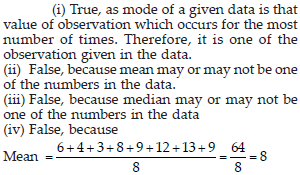Q 15.

Use the bar graph to answer the following questions.
(A) Which is the most popular pet?
(B) How many students have dog as a pet?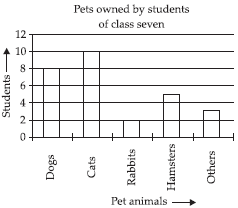SOLUTION:

(A) Since, the bar representing number of students for cats is the tallest, so cat is the most popular pet. (B) The number of students having dog as a pet are 8.

Q 16.

Read the bar graph which shows the number of books sold by a bookstore during five consecutive years and answer the following questions: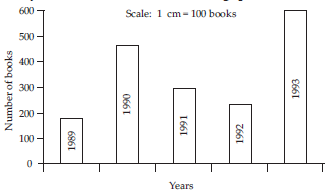(i) About how many books were sold in 1989? 1990? 1992?
(ii) In which year were about 475 books sold? About 225 books sold?
(iii) In which years were fewer than 250 books sold?
(iv) Can you explain how you would estimate the number of books sold in 1989?

SOLUTION: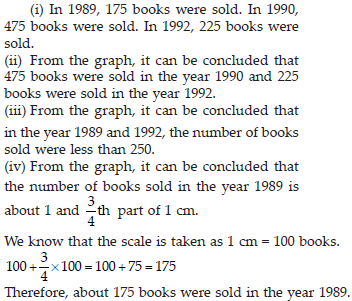Q 17.

Number of children in six different classes are given below. Represent the data on a bar graph.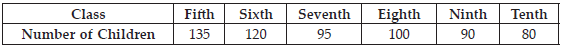(i) In which subject, has the child improved his performance the most?
(ii) In which subject is the improvement the least?
(iii) Has the performance gone down in any subject?

SOLUTION: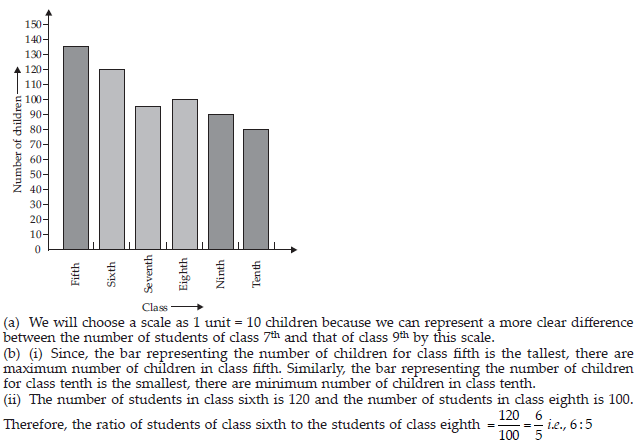Q 18.

The performance of a student in 1st Term and 2nd Term is given. Draw a double bar graph choosing appropriate scale and answer the following: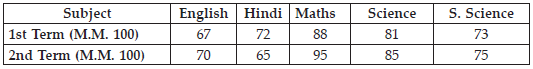(i) In which subject, has the child improved his performance the most?
(ii) In which subject is the improvement the least?
(iii) Has the performance gone down in any subject?

SOLUTION: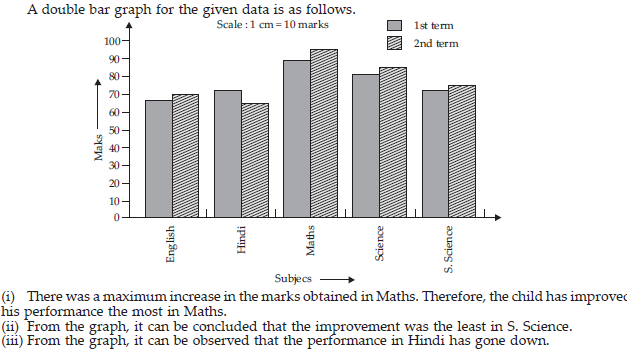Q 19.

Consider this data collected from a survey of a colony.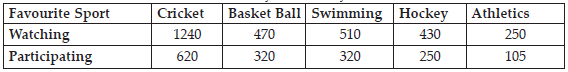(i) Draw a double bar graph choosing an appropriate scale.
What do you infer from the bar graph?
(ii) Which sport is most popular?
(iii) Which is more preferred, watching or participating in sports?

SOLUTION: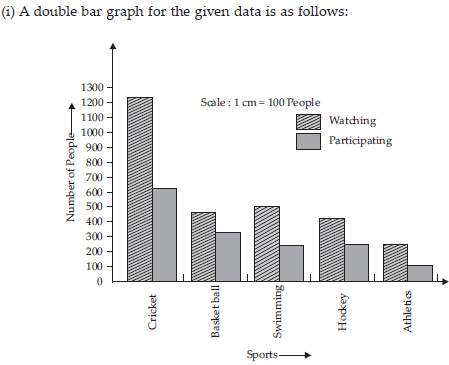The double bar graph represents he number of people who like watching and participating in sport.
(ii) From the bar graph, it can be observed that the bar representing the number of people who like watching and participating in cricket is the tallest among all the bars. Hence, cricket is the most popular sport.
(iii) The bars representing watching sport are longer than the bars representing participating in sport. Hence, watching different types of sports is more preferred than participating in the sports.

Q 20.

Take the data giving the minimum and the maximum temperature of various cities given in the table. Plot a double bar graph using the data and answer the following: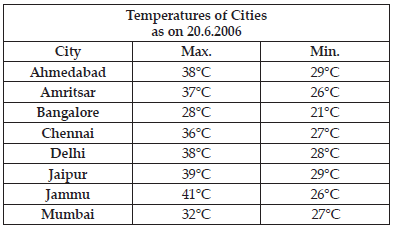(i) Which city has the largest difference in the minimum and maximum temperature on the given date?
(ii) Which is the hottest city and which is the coldest city?
(iii) Name two cities where maximum temperature of one was less than the minimum temperature of the other.
(iv) Name the city which has the least difference between its minimum and the maximum temperature.

SOLUTION: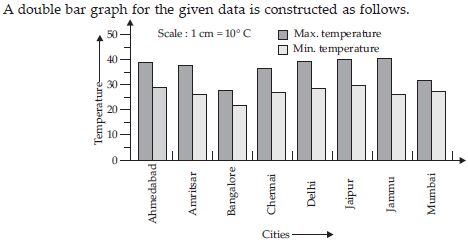(i) From the graph, it can be concluded that Jammu has the largest difference in its minimum and maximum temperatures on 20.6.2006.
(ii) From the graph, it can be concluded that Jammu is the hottest city and Bangalore is the coldest city.
(iii) Bangalore and Jaipur, Bangalore and Ahmedabad For Bangalore, the maximum temperature was 28°C, while minimum temperature of both cities, Ahmedabad and Jaipur, was 29°C
(iv) From the graph, it can be concluded that the city which has least difference between its minimum and maximum temperatures is Mumbai.

Q 21.

Tell whether the following is certain to happen, impossible, can happen but not certain.
(i) You are older today than yesterday.
(ii) A tossed coin will land heads up.
(iii) A die when tossed shall land up with 8 on top.
(iv) The next traffic light seen will be green.
(v) Tomorrow will be a cloudy day.

SOLUTION:

(i) Certain
(ii) Can happen but not certain
(iii) Impossible as there are only six faces on a die marked as 1, 2, 3, 4, 5, 6 on it.
(iv) Can happen but not certain
(v) Can happen but not certain

Q 22.

There are 6 marbles in a box with numbers from 1 to 6 marked on each of them.
(i) What is the probability of drawing a marble with number 2?
(ii) What is the probability of drawing a marble with number 5?

SOLUTION: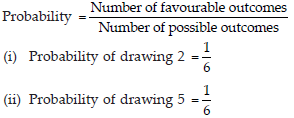Q 23.

A coin is flipped to decide which team starts the game. What is the probability that your team will start?

SOLUTION: